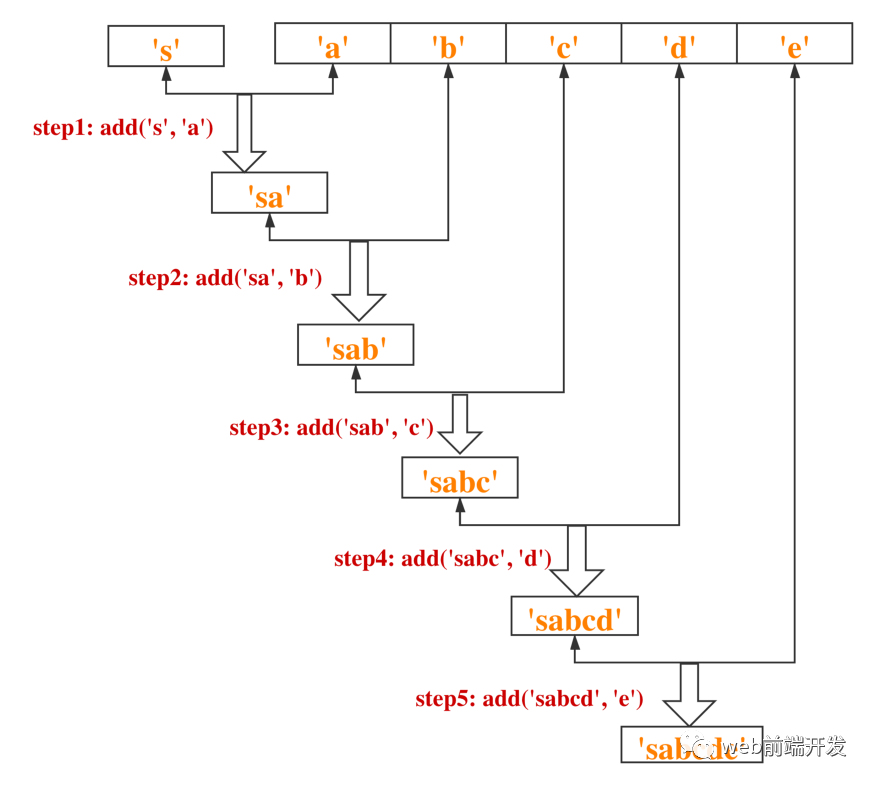# JavaScript数组reduce()方法使用实例详解

## 前言

```var arr = [1, 2, 3];
function reducer(parmar1, parmar2){
}
arr.reduce(reducer)```

reduce是数组原型对象上的一个方法，可以帮助我们操作数组。它将另一个函数作为其参数，可以称为reducer。

reducer 有两个参数。第一个参数 param1 是最后一次 reducer 运行的结果。如果这是第一次运行 reducer，则 param1 的默认值是数组第一个元素的值。

reduce 方法循环遍历数组中的每个元素，就像在 for 循环中一样。并将循环中的当前值作为参数2。

```var arr = ['a', 'b', 'c', 'd', 'e'];
return x + y;
}```var arr = ['a', 'b', 'c', 'd', 'e'];
return x + y;
}```var arr = ['a', 'b', 'c', 'd', 'e'];
return x + y;
}

## 1、累加和累积乘法

```function accumulation(arr) {
let sum = 0;
for (let i = 0; i < arr.length; i++) {
sum = sum + arr[i];
}
return sum;
}```

• 将初始总和设置为零
• 取出数组中的第一个元素并求和
• 在 sum 中缓存上一步的结果
• 依次取出数组中的其他元素，进行上述操作
• 返回最终结果

```function accumulation(arr) {
function reducer(x, y) {
return x + y
}
return arr.reduce(reducer, 0);
}```

```function accumulation(arr) {
return arr.reduce((x, y) => x + y, 0);
}``````function multiplication(arr) {
return arr.reduce((x, y) => x * y, 1);
}```

```const scores = [
{ score: 90, subject: "HTML", weight: 0.2 },
{ score: 95, subject: "CSS", weight: 0.3 },
{ score: 85, subject: "JavaScript", weight: 0.5 }
];
const result = scores.reduce((x, y) => x + y.score * y.weight, 0); // 89```

## 2、获取一个数组的最大值和最小值

```function max(arr){
let max = arr;
for (let ele of arr) {
if(ele > max) {
max = ele;
}
}
return max;
}```

```let arr = [3.24, 2.78, 999];
arr.reduce((x, y) => Math.max(x, y));
arr.reduce((x, y) => Math.min(x, y));```## 3、计算数组中元素出现的频率

```function countFrequency(arr) {
return arr.reduce(function(result, ele){
// Judge whether this element has been counted before
if (result.get(ele) != undefined) {
/**
* If this element has been counted before,
* increase the frequency of its occurrence by 1
*/
result.set(ele, result.get(ele) + 1)
} else {
/**
* If this element has not been counted before,
* set the frequency of its occurrence to 1
*/
result.set(ele, 1);
}
return result;
}, new Map());
}``````let str = 'helloworld';
str.split('').reduce((result, currentChar) => {
result[currentChar] ? result[currentChar] ++ : result[currentChar] = 1;
return result;
}, {})```## 4、多个数组的展平

```function Flat(arr = []) {
return arr.reduce((t, v) => t.concat(Array.isArray(v) ? Flat(v) : v), [])
}```## 总结

• JavaScript数组常用方法实例讲解总结
• JavaScript数组方法实例详解
• JS数组Array常用方法汇总+实例
• js判断两个数组相等的5种方法实例
• JavaScript数组reduce常见实例方法
• 梳理总结25个JavaScript数组操作方法实例
• JavaScript中array.reduce()数组方法的四种使用实例
• JavaScript数组实例的9个方法